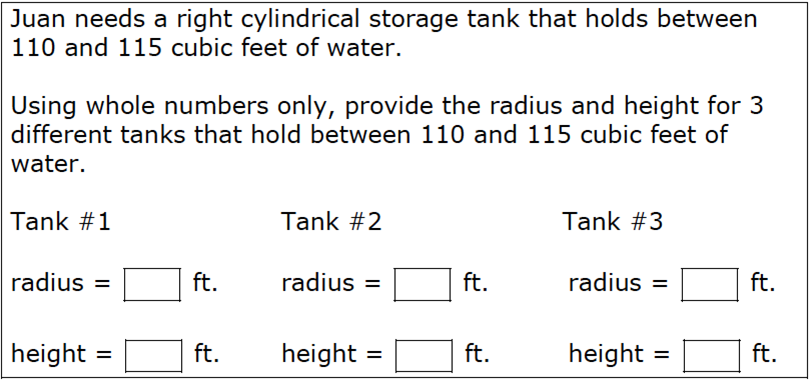# Cylinders### Hint

Is there a formula that can help us solve this problem?

When it says, “the tank holds between 110 and 115 cubic feet of water,” what mathematical concept are they referring to?

r=1 and h = 36, r = 2 and h = 9, r = 3 and h = 4, r = 6 and h = 1

## Triangle Sum Theorem

Directions: Using the digits 1-9 at most one time each, fill in the blanks so …

1.•2.3.